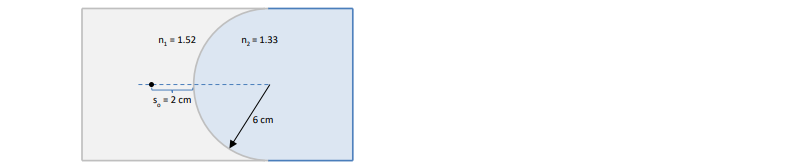Clutch Prep is now a part of Pearson
Ch 34: Geometric OpticsWorksheetSee all chapters

# Refraction At Spherical Surfaces

See all sections
Sections
Ray Nature Of Light
Reflection Of Light
Refraction Of Light
Total Internal Reflection
Ray Diagrams For Mirrors
Mirror Equation
Refraction At Spherical Surfaces
Ray Diagrams For Lenses
Thin Lens And Lens Maker Equations

Concept #1: Refraction at Spherical Surfaces

Transcript

Hey guys, in this video we're going to talk about refraction and image formation at spherical surfaces we saw before when we talked about Snell law how to apply refraction to a single ray of light across a flat boundary now we want to look at what happens when multiple rays of light come from an object refract through a spherical boundary and form an image inside of a different medium let's get to it.A single ray of light passing through a transparent surface undergoes refraction all right this is something we know we know how to apply Snell's law to figure this out but many rays of light will also undergo refraction together. An object placed in front of a surface a transparent surface that allows transmission of light will form an image based on the focal length that surface has those images can be real or virtual based on the shape of the surface so that surface can have a positive focal length or it can have a negative focal length all right the image distance equation for a spherical surface is this. Where N1 is the index of refraction that the object is in and N2 is the index of refraction across the boundary that the light rays are passing into now there are some sign conventions that are important for this equation first for a convex surface like the one in the image above me that I scroll down past the radius is always considered positive. When you plug it into this equation, For concave surfaces the radius is considered negative and just like before just like we had for mirrors if you calculate a positive image distance that is a real image that is inverted and if you calculate a negative image distance that's a virtual image that is upright so this is exactly the same as it was for mirrors. Let's do an example an object in air is placed 5 centimeters in front of a transparent concave surface if the radius of curvature is 7 centimeters and the refractive index behind the surface is 1.44 where is the image located is the image real or virtual because this is a single surface refraction we want to use our equation for that N1 over the object distance plus N2 over the image distance equals the difference between those indices divided by the radius now because this is a concave surface the radius is going to be negative remember that's one of our rules and it's important to remember the sign convention. What we have is our initial index of refraction which our initial medium is air is 1. Our object distance was told is 5 centimetres in front of the surface so it's 5 centimetres.The index of refraction behind the boundary is 1.44.Our image distance we don't know and finally our radius of curvature is -7 centimetres it's important to remember that negative sign because if you don't the answer is going to be completely off it's not just going to be off by a sign. So let's rearrange this equation to get N2 over SI equals N2 minus N1 over R minus N1 over SO and let's plug in those numbers this is going to be 1.44 minus 1 over -7 minus 1 over positive 5.Which if you plug into your calculator equals - 0.263. Let me minimise myself let me get myself just a little bit more space here so we have N2 over S.I equals - 0.263.If I multiply the S.I up and divide by -0.263 I get S.I is N2 over a -0.263 the second refractive index is 1.44 and this answer is -5.5 centimetres.Very simple to just apply the equation even though the arithmetic can get a little bit hairy now is this image real or virtual don't forget the sign convention for images if it's a negative image distance it is virtual.The question didn't ask for it but the image is also upright because virtual images are always upright alright guys that wraps up our discussion on refraction at a single surface a single spherical surface. Thanks for watching guys.

Practice: An object is embedded in glass as shown in the following figure. If the glass has a concave face, and is embedded in water, where will the image be located? Will the image be real or virtual?